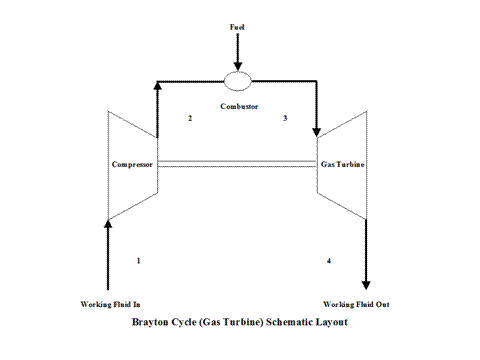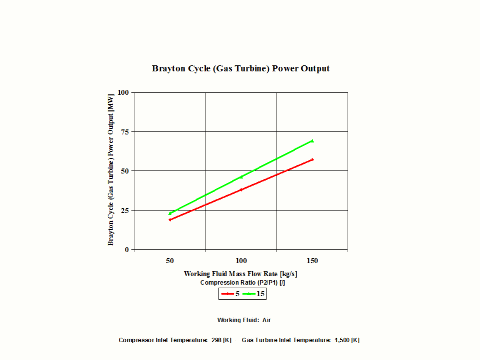top of page

### Brayton Cycle (Power) Analysis

Brayton Cycle -- Power Application

Introduction

This section provides a Brayton Cycle analysis when the working fluid is air.

Analysis

In the presented Brayton Cycle analysis, only air is considered as the working fluid behaving as a perfect gas -- specific heat has a constant value.  Ideal gas state equation is valid -- pv = RT.

A gas turbine is a heat engine that uses a high temperature, high pressure gas as the working fluid.  Combustion of a fuel in air is usually used to produce the needed temperatures and pressures in the gas turbine, which is why gas turbines are often referred to as combustion turbines.  Expansion of the high temperature, high pressure working fluid takes place in the gas turbine.  The gas turbine shaft rotation drives an electric generator and a compressor for the working fluid, air, used in the gas turbine combustor.  Many gas turbines also use a heat exchanger called a recouperator to impart turbine exhaust heat into the combustor's air/fuel mixture.  Gas turbines produce high quality heat that can be used to generate steam for combined heat and power and combined-cycle applications, significantly enhancing efficiency.

The initial state of the working fluid, air, is assumed to be at point 1 at the atmospheric conditions, 298 [K] temperature and 1 [atm] pressure.  Air is compressed, isentropically, along line 1-2 by a compressor and enters a combustor.  At a constant pressure, combustion takes place (fuel is added to the combustor and the air temperature raises) and/or heat gets added to air.  High temperature air exits the combustor at point 3.  Then air enters a gas turbine where an isentropic expansion occurs, producing power.  Air exits the gas turbine at point 4.  It should be mentioned that air at point 1 enters the compressor and the cycle is repeated.

A schematic layout of the ideal, open Brayton Cycle is shown in Figure 1.Figure 1 - Brayton Cycle Schematic Layout

Figure 2 presents a Brayton Cycle temperature vs entropy diagram.Figure 2 - Brayton Cycle Temperature vs Entropy Diagram

It should be pointed out that this material deals with the open Brayton Cycle.  The way how the T - s diagram is presented, it describes a closed Brayton Cycle -- this would require a heat exchanger after point 4 where the working fluid would be cooled down to point 1 and the cycle repeats.  Therefore, the T - s diagram is presented as a closed Brayton Cycle to allow easier understanding and derivation of the Brayton Cycle thermal efficiency -- heat addition and heat rejection.

The gas turbine and compressor are connected by shaft so the considerable amount of work done on the gas turbine is used to power the compressor.
It can be noticed from the T - s diagram that the work done on the gas turbine is greater than the work necessary to power the compressor -- constant pressure lines in the T - s diagram diverge by going to the right side (entropy wise).

Figure 3 presents the Brayton Cycle efficiency as a function of the compression  ratio.  It should be noted that the inlet conditions are standard ambient conditions:  temperature of 298 [K] and absolute pressure of 1 [atm]. me. It's easy.Figure 3 - Brayton Cycle Efficiency

Here, two general performance trends are considered.  First, impact of the gas turbine inlet temperature and compression ratio values on the Brayton Cycle specific power output and second, impact of the working mass flow rate for a fixed gas turbine inlet temperature on the Brayton Cycle power output.

Figure 4 presents the results of the first performance trend, while Figure 5 presents the results of the second trend.Figure 4 - Brayton Cycle Specific Power OutputFigure 5 - Brayton Cycle Power Output

One can notice that the Brayton Cycle efficiency increases with an increase in the compression ratio values.  One can notice that the Brayton Cycle specific power output increases with an increase in the gas turbine inlet temperature.  Furthermore, the increase is greater for the higher compression ratio values.

One can notice that the Brayton Cycle power output increases with an increase in the working fluid mass flow rate for a fixed gas turbine inlet temperature.  The increase is greater for the higher compression ratio values.

Assumptions

Working fluid is air.  There is no friction.  Compression and expansion processes are reversible and adiabatic -- isentropic.  Ideal gas state equation is valid -- pv = RT.  Air behaves as a perfect gas --specific heat has a constant value.

Governing Equations

T2/T1 = (p2/p1)^(ϰ-1)/ϰ
p
2/p1 = (T2/T1)^
ϰ/(ϰ-1)
T
3/T4 = (p3/p4)^(
ϰ-1)/ϰ
p
3/p4 = (T3/T4)^
ϰ/(ϰ-1)
ϰ = cp/cv
cp - cv = R
pv = RT
w = q
h - q
qh = cp(T3 - T2
q
l = cp(T4 - T1
w = c
p(T3 - T2) - cp(T4 - T1
W = (c
p(T3 - T2) - cp(T4 - T1))m
efficiency = 1 - 1/r
p^(
ϰ-1)/ϰ
r
p = p2/p1

Input Data

T1 = 298 [K]

p1 = 1 [atm]
T
3 = 900, 1,200 and 1,500 [K]

p3 = 5, 10, 15, 20 and 25 [atm]
R = 0.2867 [kJ/kg*K]
c
p = 1.004 [kJ/kg*K]

ϰ = 1.4 [/]

m = 50, 100 and 150 [kg/s]

Results

Brayton Cycle Efficiency vs Compression Ratio

Compression
Ratio
[/]

5

10

15

20

25

Brayton Cycle
Efficiency
[%]

36.92

48.22

53.87

57.53

60.16

Specific Power Output vs Compression Ratio
for a few Gas Turbine Inlet Temperature Values

Specific Power Output
[kW/kg/s]

Compression Ratio
[/]

5

15

Gas Turbine Inlet
Temperature
[K]

900

159

137

1,200

270

300

1,500

381

462

Power Output vs Compression Ratio
for a few Mass Flow Rates
Gas Turbine Inlet Temperature = 1,500 [K]

Power Output
[MW]

Compression Ratio
[/]

5

15

Mass Flow Rate
[kg/s]

50

19.1

23.1

100

38.1

46.2

150

57.2

69.3

Conclusions

The Brayton Cycle efficiency depends on the compression ratio and working fluid properties.  The efficiency increases with an increase in the compression ratio values.  Also, the efficiency increases with the higher value for kappa, ϰ, which is a ratio of gas specific heat values (cp/cv).

The Brayton Cycle specific power output increases with an increase in the gas turbine inlet temperature.  Furthermore, the increase is greater for the higher compression ratio values.  The Brayton Cycle power output increases with an increase in the working fluid mass flow rate for a fixed gas turbine inlet temperature.  The increase is greater for the higher compression ratio values.

References

JANAF Thermochemical Data - Tables, 1970

bottom of page